Courses

# Electric Field And Potential MCQ Level - 1

## 10 Questions MCQ Test Basic Physics for IIT JAM | Electric Field And Potential MCQ Level - 1

Description
This mock test of Electric Field And Potential MCQ Level - 1 for Physics helps you for every Physics entrance exam. This contains 10 Multiple Choice Questions for Physics Electric Field And Potential MCQ Level - 1 (mcq) to study with solutions a complete question bank. The solved questions answers in this Electric Field And Potential MCQ Level - 1 quiz give you a good mix of easy questions and tough questions. Physics students definitely take this Electric Field And Potential MCQ Level - 1 exercise for a better result in the exam. You can find other Electric Field And Potential MCQ Level - 1 extra questions, long questions & short questions for Physics on EduRev as well by searching above.
QUESTION: 1

### Let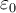denote the permittivity of vacuum, the dimensional formula of the ε0 is  let M = Mass, L = length, T = time and I = electric current. Then

Solution:

Using Coulomb law, F = 1/4πϵ0(q1q2/R2)

⇒ϵ0 = q1q2/4πFR2

Substituting the units in the equation:

So, ϵ0 = C2/N.m2

=[AT]2/MLT-2.L2

=[M-1L-3T4A2]

QUESTION: 2

### A charge q1 exert some force on a second charge q2. If a third charge q3 is brought near q1 the force exerted by q1 on q2

Solution:

The correct answer is: Remain the same

QUESTION: 3

### An electric dipole is place in a uniform electric field. The net electric force on the dipole

Solution: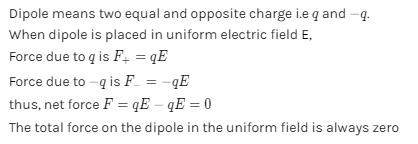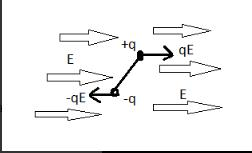QUESTION: 4

Which of the following is not true?

Solution:

The correct answer is: The electric dipole potential varies as 1/r at large distance

QUESTION: 5

A charge particle is free to move in an electric field. It will travel

Solution:

The correct answer is: Along a line of force, if its initial velocity is zero

QUESTION: 6

A uniform electric field of 100 V/m is directed at 30° with the positive x-axis as shown in figure. Find the potential difference VBA. If  OA = 2m and OB = 4m. O is the origin.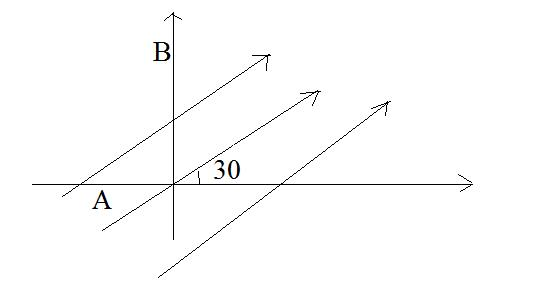Solution:

Electric field in vector form can be written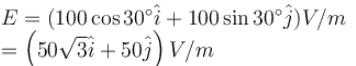A = (–2m, 0, 0)
B = (0.4m, 0)
VAB = VB – VA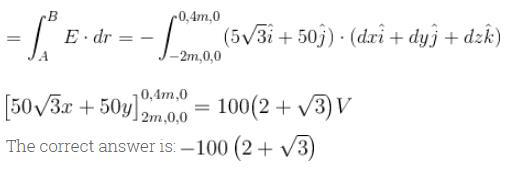QUESTION: 7

The curve represents the distribution of potential along the straight line joining. The two charge Q1 and Q2 (separated by a distance r) then which of the following statement is correct?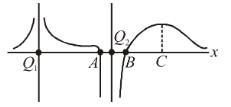(1)  |Q1| > |Q2|
(2) Q1 is positive in nature
(3)  A and B are equilibrium

Solution:

The correct answer is: 1, 2 and 3

QUESTION: 8

The number of electrons to be put on a spherical conductor of radius 0.1m to produce on electric field of 0.036NC-1 just above its surface is

Solution: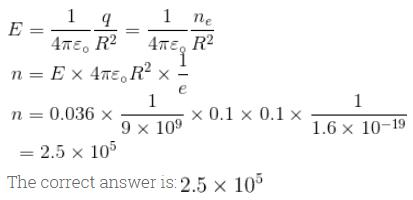QUESTION: 9

Proton is nearly 1836 times more massive than the electron. The coulomb force of repulsion between two proton separated by a finite distance is F. The electrostatic force between two electron separated by the same distance is

Solution:

Coulomb force is determined by charges not by masses.